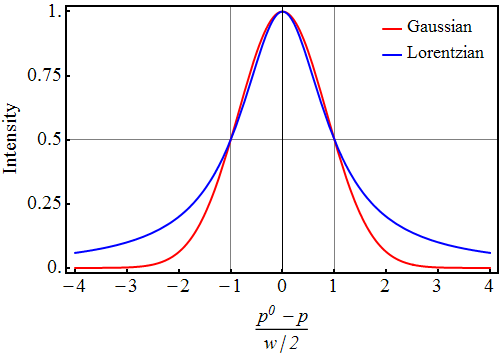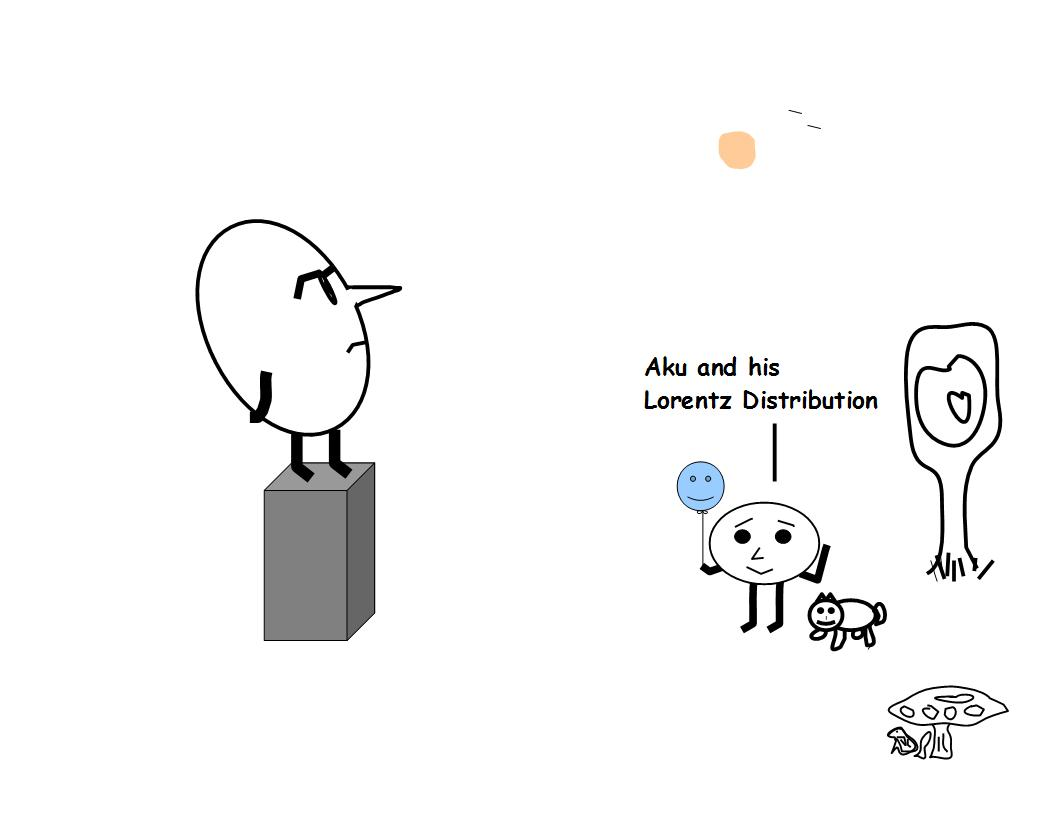# ijspace.org

 Cauchy and Gaussian Distributions 20th April 2014          Cauchy or Lorentz distribution is used to describe the characteristics of atomic and molecular spectra.  It has no defined mean or variance as the curve never touches the horizontal axis.We also have Gaussian or normal distribution which is used extensively to describe statistical phenomena.  For example if we were to describe a phenomenon based on temperature only, we can use the normal distribution.  However if we were to include either interactions or Doppler broadening, the Lorentz distribution is more appropriate.  To simplify, the steady-state assumption would lead to the Normal distribution.  However the real picture is represented by Cauchy or Lorentz distribution.       If we are measuring an infinite source then steady-state can not be assumed.  The steady-state can be assumed iff the initial state is disconnected by an assumption of a symmetry.  The Normal distribution will shift towards Cauchy, if more and more interaction is included in the description.  Vice versa a Cauchy distribution will move towards Gaussian, as the system gets more and more isolated.       The Lorentz distribution is given as f(x) = 1/π(1+x2).  The Lorentz distribution has no poles in real plane and its mathematical properties can be found in the literature.  What we would like to point out is that the situation regarding poles changes when we consider the Lorentz distribution in complex plane i.e. f(z) = 1/π(1+z2).  In that case the poles are found at z = +i and z = -i.  Important is to think what does the complex plane represent in terms of observer and his/her measurements?       In nature the physical examples of the Lorentz distribution are the cross-sections of a single water wave, and of a smooth hill.  The water wave is formed at the water-wind interface where the nature of force is largely tangential.  The normal component is also present and it determines the depth to which the applied force penetrates.  Further the area affected by the force is infinitesimal with respect to the content of the medium being affected.  Thus the Lorentz distribution represents the structures measured when the strength of the applied force is very small compared to the strength of the medium.       So it all leads back to the significance of the fine-structure constant, such an important parameter in spectroscopy and QED.  What does it really represent? The electron-photon interactions most certainly do not provide the complete picture.  This we have already shown that we are in the discrete j-space when it comes to measurements.  But the question remains that can we ever measure a significant portion of the information that is available?  Highly unlikely without a monumental change in our way of thinking about nature.       Our thanks to RPG for mentioning the significance of Lorentz Distribution in spectroscopy.Information on www.ijspace.org is licensed under a Creative Commons Attribution 4.0 International License.